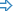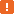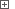When testing an application that uses DataGridView controls, you may need to search for a specific record within the grid. For example, you may need to find a certain record to make sure that the data has been loaded into the grid correctly or to determine the position of a record within the grid. This topic describes two approaches for searching within the DataGridView control and explains how you can implement a custom search:Searching Within the Grid’s DatasetSearching Within the GridTo perform these actions, TestComplete should have access to internal objects, properties and methods of the DataGridView control. For this purpose, the .NET Application Support and Microsoft Control Support plugins must be installed and enabled.When testing Microsoft DataGridView controls, use specific methods and properties of the corresponding `MicrosoftDataGridView` object. You can call these methods and properties from your keyword tests, as well as from scripts. This topic describes how to work with an object’s properties and methods from your scripts. However, when testing a DataGridView control from your keyword test, you can use the same methods and properties calling them from keyword test operations. For more information, see Keyword Tests Basic Operations.
##### Searching Within the Grid’s Dataset

If the DataGridView control is bound to an external dataset, you can search for the desired record within the dataset rather than within the grid itself. Searching within the grid’s dataset is a universal approach that is free from the grid’s user interface limitations. You can also use this approach if you need to obtain and process values in the grid cells rather than their displayed representation.

To obtain the grid’s underlying dataset, use the `DataSource` property of the grid control. Since the DataGridView control can be bound to various source types, the object returned by the `DataSource` property may have a different structure in different applications. To explore the dataset object, find the grid’s `DataSource` property in the Object Browser panel and click the ellipsis button on the right of the property cell -- you will see the properties and methods of the object returned by this property. Digging further, you will be able to determine the exact “path” to the table object containing the data and the methods that can be used to search within this table.

Let’s illustrate this approach by the example of the grid bound to the ADO.NET dataset. You can search in the dataset using the `Find` or `FindRows` method of a .NET `DataView` object that represents a view of the grid data. To obtain the `DataView` object, you can use this statement:

GridObj.BindingContext.Item_2( GridObj.DataSource, GridObj.DataMember ).List

To search within the `DataView` object, you can use the `Find` or `FindRows` methods. These methods search in the column of the currently sorted grid. The difference between these methods is that `Find` returns the index of the first found row that matches the search criteria, whereas `FindRows` returns the array of objects corresponding to all matching rows. The parameter of both methods is the value that is sought for (integer, double, string, date or any other). To search for a complex value (such as `System.DateTime`), you need to create an instance of the corresponding .NET class (see Calling Functions From .NET Assemblies). If rows that match the search criteria are not found, `Find` returns -1 and `FindRows` returns an empty array (with no elements).

Below is an example that demonstrates how you can search for a grid row using the `DataView.Find` method.

###### ExampleView description

JavaScript, JScript

function Main ()
{
var p, Grid, RowIndex;

// Obtain the grid object
p = Sys.Process ("DataGridViewSample");
Grid = p.WinFormsObject("Form1").WinFormsObject("dataGridView1");

// Sort the grid data by the "Customer Name" column

// Locate a row by cell value
RowIndex = FindRowInDataset (Grid, "Samuel Clemens");
if (RowIndex >= 0)
Grid.ClickCell (RowIndex, "Customer Name");
else
}

function FindRowInDataset (Grid, Value)
{
// Get the grid's DataView
var DataView = Grid.BindingContext.Item_2(Grid.DataSource, Grid.DataMember).List;

// Find data row containing the specified value
return DataView.Find (Value);
}

Python

``````def Main ():

# Obtain the grid object
p = Sys.Process ("DataGridViewSample")
Grid = p.WinFormsObject("Form1").WinFormsObject("dataGridView1")

# Sort the grid data by the "Customer Name" column

# Locate a row by cell value
RowIndex = FindRowInDataset (Grid, "Samuel Clemens")
if (RowIndex >= 0):
Grid.ClickCell (RowIndex, "Customer Name")
else:

def FindRowInDataset (Grid, Value):
# Get the grid's DataView
DataView = Grid.BindingContext.Item_2[Grid.DataSource, Grid.DataMember].List

# Find data row containing the specified value
return DataView.Find (Value)``````

VBScript

Sub Main
Dim p, Grid, RowIndex

' Obtain the grid object
Set p = Sys.Process ("DataGridViewSample")
Set Grid = p.WinFormsObject("Form1").WinFormsObject("dataGridView1")

' Sort the grid data by the "Customer Name" column

' Locate a row by cell value
RowIndex = FindRowInDataset (Grid, "Samuel Clemens")
If RowIndex >= 0 Then
Call Grid.ClickCell (RowIndex, "Customer Name")
Else
End If
End Sub

Function FindRowInDataset (Grid, Value)
Dim DataView
' Get the grid's DataView
Set DataView = Grid.BindingContext.Item_2(Grid.DataSource, Grid.DataMember).List.List

' Find data row containing the specified value
FindRowInDataset = DataView.Find (Value)
End Function

DelphiScript

function FindRowInDataset (Grid, Value);
var DataView : OleVariant;
begin
// Get the grid's DataView
DataView := Grid.BindingContext.Item_2[Grid.DataSource, Grid.DataMember].List.List;

// Find data row containing the specified value
Result := DataView.Find (Value);
end;

procedure Main;
var p, Grid, RowIndex : OleVariant;
begin
// Obtain the grid object
p := Sys.Process ('DataGridViewSample');
Grid := p.WinFormsObject('Form1').WinFormsObject('dataGridView1');

// Sort the grid data by the 'Customer Name' column

// Locate a row by cell value
RowIndex := FindRowInDataset (Grid, 'Samuel Clemens');
if RowIndex >= 0 then
Grid.ClickCell (RowIndex, 'Customer Name')
else
end;

C++Script, C#Script

function Main ()
{
var p, Grid, RowIndex;

// Obtain the grid object
p = Sys["Process"]("DataGridViewSample");
Grid = p["WinFormsObject"]("Form1")["WinFormsObject"]("dataGridView1");

// Sort the grid data by the "Customer Name" column

// Locate a row by cell value
RowIndex = FindRowInDataset (Grid, "Samuel Clemens");
if (RowIndex >= 0)
Grid["ClickCell"](RowIndex, "Customer Name");
else
}

function FindRowInDataset (Grid, Value)
{
// Get the grid's DataView
var DataView = Grid["BindingContext"]["Item_2"](Grid["DataSource"], Grid["DataMember"])["List"];

// Find data row containing the specified value
return DataView["Find"](Value);
}

##### Searching Within the Grid

If the DataGridView control in the tested application works in the unbound mode or if it is bound to a custom source, you should search within the grid itself rather that within its dataset. You should also search within the grid if it is configured so that it displays values with a custom formatting applied.

The DataGridView control does not have built-in methods for searching within the grid, so you will need to implement the search algorithm yourself. A general search approach implies that you iterate through the grid rows and on each iteration check whether the row meets the specified condition. For more information on how to iterate through the grid, see Iterating Through Rows in Microsoft DataGridView.

Below is an example that demonstrates how you can locate a particular row by iterating through the grid rows.

###### ExampleView description

JavaScript

function Main ()
{
var p, Grid, RowIndex;

// Obtain the grid object
p = Sys.Process ("DataGridViewSample");
Grid = p.WinFormsObject("Form1").WinFormsObject("dataGridView1");

// Locate a row by cell value
RowIndex = FindRow (Grid, "Customer Name", "Samuel Clemens");
if (RowIndex >= 0)
Grid.ClickCell (RowIndex, "Customer Name");
else
}

function FindRow (Grid, ColumnId, Value)
{
// Iterate through grid rows
for (let i=0; i<Grid.wRowCount; i++)
// Check the cell value in the specified column
if (equal(Grid.wValue(i, ColumnId), Value))
return i;  // Row is found

}

JScript

function Main ()
{
var p, Grid, RowIndex;

// Obtain the grid object
p = Sys.Process ("DataGridViewSample");
Grid = p.WinFormsObject("Form1").WinFormsObject("dataGridView1");

// Locate a row by cell value
RowIndex = FindRow (Grid, "Customer Name", "Samuel Clemens");
if (RowIndex >= 0)
Grid.ClickCell (RowIndex, "Customer Name");
else
}

function FindRow (Grid, ColumnId, Value)
{
// Iterate through grid rows
for (var i=0; i<Grid.wRowCount; i++)
// Check the cell value in the specified column
if (Grid.wValue(i, ColumnId) == Value)
return i;  // Row is found

}

Python

``````def Main ():

# Obtain the grid object
p = Sys.Process ("DataGridViewSample")
Grid = p.WinFormsObject("Form1").WinFormsObject("dataGridView1")

# Locate a row by cell value
RowIndex = FindRow (Grid, "Customer Name", "Samuel Clemens")
if (RowIndex >= 0):
Grid.ClickCell (RowIndex, "Customer Name")
else:

def FindRow (Grid, ColumnId, Value):
# Iterate through grid rows
for i in range(0, Grid.wRowCount-1):
# Check the cell value in the specified column
if (Grid.wValue[i, ColumnId] == Value):
return i  # Row is found

VBScript

Sub Main
Dim p, Grid, RowIndex

' Obtain the grid object
Set p = Sys.Process ("DataGridViewSample")
Set Grid = p.WinFormsObject("Form1").WinFormsObject("dataGridView1")

' Locate a row by cell value
RowIndex = FindRow (Grid, "Customer Name", "Samuel Clemens")
If RowIndex >= 0 Then
Call Grid.ClickCell (RowIndex, "Customer Name")
Else
End If
End Sub

Function FindRow (Grid, ColumnId, Value)
Dim i
' Iterate through grid rows
For i = 0 To Grid.wRowCount-1
' Check the cell value in the specified column
If Grid.wValue(i, ColumnId) = Value Then
FindRow = i  ' Row is found
Exit Function
End If
Next

End Function

DelphiScript

function FindRow (Grid, ColumnId, Value);
var i : OleVariant;
begin
// Iterate through grid rows
for i :=0 to Grid.wRowCount-1 do
// Check the cell value in the specified column
if Grid.wValue[i, ColumnId].Equals(Value) then
begin
Result := i;  // Row is found
Exit
end;

end;

procedure Main;
var p, Grid, RowIndex : OleVariant;
begin
// Obtain the grid object
p := Sys.Process ('DataGridViewSample');
Grid := p.WinFormsObject('Form1').WinFormsObject('dataGridView1');

// Locate a row by cell value
RowIndex := FindRow (Grid, 'Customer Name', 'Samuel Clemens');
if RowIndex >= 0 then
Grid.ClickCell (RowIndex, 'Customer Name')
else
end;

C++Script, C#Script

function Main ()
{
var p, Grid, RowIndex;

// Obtain the grid object
p = Sys["Process"]("DataGridViewSample");
Grid = p["WinFormsObject"]("Form1")["WinFormsObject"]("dataGridView1");

// Locate a row by cell value
RowIndex = FindRow (Grid, "Customer Name", "Samuel Clemens");
if (RowIndex >= 0)
Grid["ClickCell"](RowIndex, "Customer Name");
else
}

function FindRow (Grid, ColumnId, Value)
{
// Iterate through grid rows
for (var i=0; i<Grid["wRowCount"]; i++)
// Check the cell value in the specified column
if (Grid["wValue"](i, ColumnId) == Value)
return i;  // Row is found

}

The following example is a bit more complex. It demonstrates how to find a DataGridView row by values held in multiple cells.

###### ExampleView description

JavaScript, JScript

function Main ()
{
var p, Grid, ColumnIds, Values, RowIndex;

// Obtain the grid object
p = Sys.Process ("DataGridViewSample");
Grid = p.WinFormsObject("Form1").WinFormsObject("dataGridView1");

// Locate a row by multiple values
ColumnIds = new Array ("Customer Name", "Product");
Values = new Array ("Susan McLaren", "MyMoney");
RowIndex = FindRowByMultipleValues (Grid, ColumnIds, Values);
if (RowIndex >= 0)
Grid.ClickCell (RowIndex, "Customer Name");
else
}

function FindRowByMultipleValues (Grid, Columns, Values)
{
var match, i, j;

// Iterate through grid rows
for (i=0; i<Grid.wRowCount; i++)
{
match = true;

// Check cell values in the specified columns
for (j=0; j<Columns.length; j++)
if (Grid.wValue(i, Columns[j]) != Values[j])
{
match = false; // Cell value differs from the specified one
break;
}

if (match)
return i;  // Row is found
}

}

Python

``````def Main ():

# Obtain the grid object
p = Sys.Process ("DataGridViewSample")
Grid = p.WinFormsObject("Form1").WinFormsObject("dataGridView1")

# Locate a row by multiple values
ColumnIds = list("Customer Name", "Product")
Values = list("Susan McLaren", "MyMoney")
RowIndex = FindRowByMultipleValues (Grid, ColumnIds, Values)
if (RowIndex >= 0):
Grid.ClickCell (RowIndex, "Customer Name")
else:

def FindRowByMultipleValues (Grid, Columns, Values):

# Iterate through grid rows
for i in range(0, Grid.wRowCount-1):
match = True

# Check cell values in the specified columns
for j in range(0, Columns.length-1):
if (Grid.wValue[i, Columns[j]] != Values[j]):
match = False # Cell value differs from the specified one
break

if (match):
return i  # Row is found

VBScript

Sub Main
Dim p, Grid, ColumnIds, Values, RowIndex

' Obtain the grid object
Set p = Sys.Process ("DataGridViewSample")
Set Grid = p.WinFormsObject("Form1").WinFormsObject("dataGridView1")

' Locate a row by multiple values
ColumnIds = Array ("Customer Name", "Product")
Values = Array ("Susan McLaren", "MyMoney")
RowIndex = FindRowByMultipleValues (Grid, ColumnIds, Values)
If RowIndex >= 0 Then
Call Grid.ClickCell (RowIndex, "Customer Name")
Else
End If
End Sub

Function FindRowByMultipleValues (Grid, Columns, Values)
Dim match, i, j

' Iterate through grid rows
For i=0 To Grid.wRowCount-1
match = True

' Check cell values in the specified columns
For j = 0 To UBound(Columns)
If Grid.wValue(i, Columns(j)) <> Values(j) Then
match = False' Cell value differs from the specified one
Exit For
End If
Next

If match Then
FindRowByMultipleValues = i  ' Row is found
Exit Function
End If
Next

End Function

DelphiScript

function FindRowByMultipleValues (Grid, Columns, Values);
var match, i, j : OleVariant;
begin
// Iterate through grid rows
for i := 0 to Grid.wRowCount-1 do
begin
match := true;

// Check cell values in the specified columns
for j := 0 to VarArrayHighBound(Columns, 1) do
if not Grid.wValue[i, Columns[j]].Equals(Values[j]) then
begin
match := false; // Cell value differs from the specified one
Break
end;

if match then
begin
Result := i;  // Row is found
Exit
end
end;

end;

procedure Main;
var p, Grid, ColumnIds, Values, RowIndex : OleVariant;
begin
// Obtain the grid object
p := Sys.Process ('DataGridViewSample');
Grid := p.WinFormsObject('Form1').WinFormsObject('dataGridView1');

// Locate a row by multiple values
ColumnIds := CreateVariantArray (0, 1);
Values := CreateVariantArray (0, 1);
ColumnIds := 'Customer Name'; ColumnIds := 'Product';
Values := 'Susan McLaren'; Values := 'MyMoney';
RowIndex := FindRowByMultipleValues (Grid, ColumnIds, Values);
if RowIndex >= 0 then
Grid.ClickCell (RowIndex, 'Customer Name')
else
end;

C++Script, C#Script

function Main ()
{
var p, Grid, ColumnIds, Values, RowIndex;

// Obtain the grid object
p = Sys["Process"]("DataGridViewSample");
Grid = p["WinFormsObject"]("Form1")["WinFormsObject"]("dataGridView1");

// Locate a row by multiple values
ColumnIds = new Array ("Customer Name", "Product");
Values = new Array ("Susan McLaren", "MyMoney");
RowIndex = FindRowByMultipleValues (Grid, ColumnIds, Values);
if (RowIndex >= 0)
Grid["ClickCell"](RowIndex, "Customer Name");
else
}

function FindRowByMultipleValues (Grid, Columns, Values)
{
var match, i, j;

// Iterate through grid rows
for (i=0; i<Grid["wRowCount"]; i++)
{
match = true;

// Check cell values in the specified columns
for (j=0; j<Columns["length"]; j++)
if (Grid["wValue"](i, Columns[j]) != Values[j])
{
match = false; // Cell value differs from the specified one
break;
}

if (match)
return i;  // Row is found
}